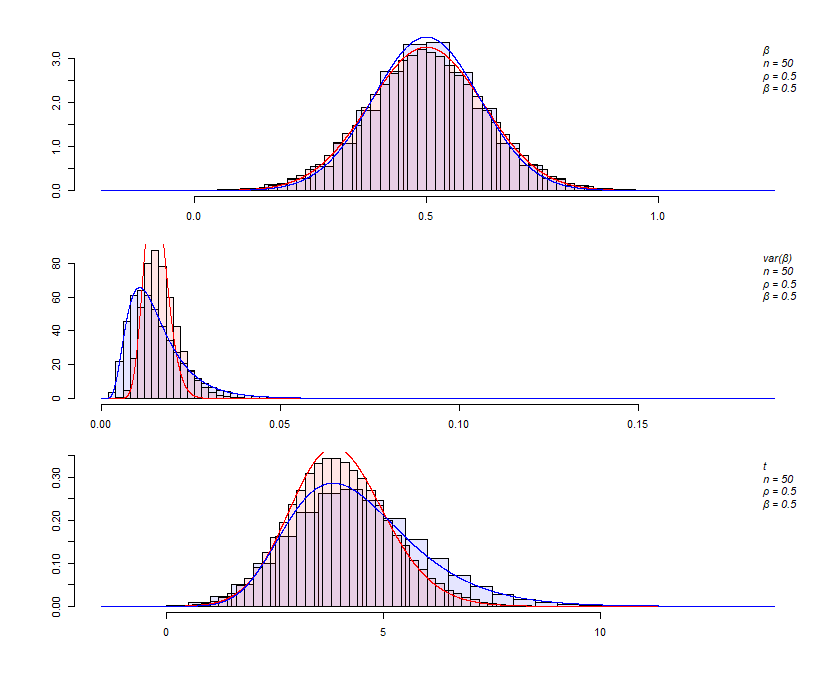# #StackBounty: #r #regression #normal-distribution #lognormal-distribution #t-distribution What are the sampling distributions underlyin…

### Bounty: 250

I’m trying for a while now to understand the differential power of main effects (two random variables) and moderated effects (the product of these two random variables). At this point, I’m mostly interested in Gaussian. I’m working with a model like :
$$begin{equation} y=beta_0 +beta_1 x+beta_2 z+beta_3 xz+epsilon_y end{equation}$$
where
$$begin{equation} begin{bmatrix} x \ z end{bmatrix} sim mathcal{N} left( begin{bmatrix}mu_X \ mu_Z end{bmatrix} ,,begin{bmatrix} 1 & rho \ rho & 1 end{bmatrix} right) end{equation}$$
I’m specifically looking for the sampling distribution of $$beta_3$$ compared to regression coefficients (like $$beta_1$$). Acknowledging that $$t$$-distributions should be used to test their power, I have found that there are many differences. Because $$t$$ is a normal ($$mathcal{N}$$) divided by $$sqrt{chi^2/n}$$, I investigated in this direction, and found out these differences.

First, the normal distribution of $$beta_3$$ as slightly different standard deviation than $$beta_1$$, i.e., $$sqrt{frac{sigma}{(n-8)(1+rho^2)}}$$ (or something very similar) instead of $$sqrt{ frac{ sigma }{n}}$$.

Second, the expected $$chi^2$$ seems to be a lognormal instead. It has heavier tails and is more skewed. I have not found how to derive the parameters of the log normal yet, but I’ve come across some approximations.

Finally, the $$t$$-distribution of $$beta_3$$ does not behave as expected. It has a slightly underestimated noncentral parameters ($$delta$$) and, more importantly, its variance is not the expected $$v/(v-2)(1+delta^2)-mu_1^2$$, $$v$$ being the degree of freedom and $$mu_1$$ is its first moment, except when $$delta=0$$. Using the previous points and this answer, I have tried to fit the function:

$$f(z)=frac{1}{2pisigma_xsigma_y}int_0^infty exp{ -[zy-mu_x]^2/2sigma_x^2-[log(y)-mu_y]^2/2sigma_y^2}~text dy$$

where $$Z$$ is the normal, $$Y$$ the lognormal, and dividing $$mu_y$$ and $$sigma_y$$ by 2, because I am interested in the square root of the lognormal (see this question).
However, this pdf does not yield "perfect" results (see last figure).

My questions are :

1. Is there a way to analytically derived the parameters of the
lognormal based on the informations in the models?
2. What would be the function to fit the "$$t$$-distribution" of $$beta_3$$ or how to correct the graphic presentation, or is it normal to be that way, or is the distribution actually a skewed generalized $$t$$ distribution?

Here is an example of code on what I’m trying to achieve.

``````library(foreach)
library(doParallel)
library(fitdistrplus)
cores=detectCores()
cl <- makeCluster(cores-1)
registerDoParallel(cl)

#the functon in https://stats.stackexchange.com/a/507175/102655
#Z=normal
#X=lognormal
f <- function(z,x,mz,mx,sz,sx) exp(-(z*x-mz)^2/(2*sz^2)-(log(x)-mx)^2/(2*sx^2))
f2 <- function(z,mz,mx,sz,sx) 1/(2*pi*sz*sx) * integrate(f=f,lower=0, upper=Inf,z=z,mz=mz,mx=mx,sz=sz,sx=sx,rel.tol=.Machine$$double.eps^.5)$$value

#some parameters
reps=100000
n=50
b=.50
rho=.5

txz = foreach(l = 1:reps, .combine = cbind) %dopar% {
x=rnorm(n)
z=rho*x + sqrt(1-rho^2) * rnorm(n)
e=rnorm(n)
y1=b*x*z+sqrt(1-b^2*(1+rho^2))*e
txz = summary(lm(y1~x*z))$$coefficient[4,1:3] w=rnorm(n) y2 = w*b+sqrt(1-b^2)*e tw = summary(lm(y2~x+z+w))$$coefficient[4,1:3]
#X = cbind(x,z,x*z)
txz = c(txz,tw)
}

Beta = matrix(c(0,0,b))
Sw = matrix(c(1,rho,0,rho,1,0,0,0,1),3,3)
Sxz = matrix(c(1,rho,0,rho,1,0,0,0,1+rho^2),3,3)

#R2 for both
R2w = c(t(Beta)%*%Sw%*%Beta)
R2xz = c(t(Beta)%*%Sxz%*%Beta)

#4th moments of the product of two gaussian
v = (3*(3+14*rho^2+3*rho^4)-(1+rho^2))

#estimation of the parameters of the lognormal
est = summary(fitdist(txz[2,]^2, distr = "lnorm", method = "mle"))\$estimate
#Would like to know them a priori
sx = est #
mx = est #

#parameters for the normal
sz=sqrt(1-R2xz)/(sqrt((n-8)*(1+rho^2)))
mz=b

#graph
par(mfcol = c(3,1), mai = c(.25, 0.25, 0.25, 0.25),oma = c(4, 4, 1, 1))
aa=txz[1,]
bb=txz[4,]
ha= hist(aa,plot=FALSE,breaks=50)
hb= hist(bb,plot=FALSE,breaks=50)
plot(ha, freq=FALSE, main=NULL,ylim = (c(0,max(ha$$density,hb$$density))),xlim=c(min(ha$$breaks,hb$$breaks),max(ha$$breaks,hb$$breaks)),col=rgb(0,0,1,.1))
xx = seq(min(ha$$breaks,hb$$breaks),max(ha$$breaks,hb$$breaks),by=sum(abs(c(min(ha$$breaks,hb$$breaks),max(ha$$breaks,hb$$breaks))))/10000)
lines(xx,dnorm(xx,mean=b,sd=sqrt(1-R2w) /(sqrt(n))),col="red", lty=1)
lines(xx,dnorm(xx,mean=mz,sd=sz),col="blue",lty=1)
tex = c("u03b2",paste0("n = ",n),paste0("u03C1 = ",rho),paste0("u03b2 = ",b))
legend("topright",legend=(tex),box.lty=0,text.font=3,bg="transparent")

aa=txz[2,]^2
bb=txz[5,]^2
ha= hist(aa,plot=FALSE,breaks=75)
hb= hist(bb,plot=FALSE,breaks=25)
plot(ha, freq=FALSE, main=NULL,ylim = (c(0,max(ha$$density,hb$$density))),xlim=c(min(ha$$breaks,hb$$breaks),max(ha$$breaks,hb$$breaks)),col=rgb(0,0,1,.1))
xx = seq(min(ha$$breaks,hb$$breaks),max(ha$$breaks,hb$$breaks),by=sum(abs(c(min(ha$$breaks,hb$$breaks),max(ha$$breaks,hb$$breaks))))/10000)
y=dchisq((xx)*(n-4)*(n-1)/(1-R2w), df=(n-4))
lines(xx,y*(n-4)*(n-1)/(1-R2w),lty=1,lwd=1,col="red")
y=dlnorm(xx,meanlog=mx,sdlog=sx)
lines(xx,y,lty=1,col="blue")
tex = c("var(u03b2)",paste0("n = ",n),paste0("u03C1 = ",rho),paste0("u03b2 = ",b))
legend("topright",legend=(tex),box.lty=0,text.font=3,bg="transparent")

aa=txz[3,]
bb=txz[6,]
ha= hist(aa,plot=FALSE,breaks=50)
hb= hist(bb,plot=FALSE,breaks=75)
plot(ha,freq=FALSE, main=NULL,ylim = (c(0,max(ha$$density,hb$$density))),xlim=c(min(ha$$breaks,hb$$breaks),max(ha$$breaks,hb$$breaks)),col=rgb(0,0,1,.1))
xx = seq(min(ha$$breaks,hb$$breaks),max(ha$$breaks,hb$$breaks),by=sum(abs(c(min(ha$$breaks,hb$$breaks),max(ha$$breaks,hb$$breaks))))/10000)
yy=dt(xx,ncp=b*sqrt(n-4)/sqrt(1-R2w),df=n-2)
lines(xx,yy,col="red")
yy = apply(as.matrix(xx),FUN=f2,MARGIN=1,mz=mz,mx=mx/2,sz=sz,sx=sx/2)
lines(xx,yy,col="blue")
tex = c("t",paste0("n = ",n),paste0("u03C1 = ",rho),paste0("u03b2 = ",b))
legend("topright",legend=(tex),box.lty=0,text.font=3,bg="transparent")
``````The blue is related to $$beta_3$$ and the red to $$beta_1$$. The simulation use $$n=50, rho=.5, beta_1=beta_3=.5$$ (both $$beta$$ are used in different data sets). I am confident for the results in red histograms and red lines, but not necessarily for the blue ones.